# 在单一工具上使用不同的 EA 交易进行交易时 ORDER_MAGIC 的使用

6 十二月 2013, 10:47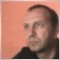1
2 073

### 简介

 类型 字节数 最小值 最大值 C + + 语言中的对等类型 long 8 -9 223 372 036 854 775 808 9 223 372 036 854 775 807 __int64 ulong 8 0 18 446 744 073 709 551 615 unsigned __int64

1000 - 仅是 3 个完整的类别，那么，对于剩下的 15 个可采用类型 ulong 的完整类别，我们应该怎么办？答案十分简单：对它们进行编码。

1. 两个或更多 EA 交易在一个工具上工作且互不干扰的可能性。
2. 两个或更多 EA 交易在不同工具上工作且相互补充的可能性。
3. 使用 EA 交易按工具识别委托的能力。

```//+------------------------------------------------------------------
//| 这个类提供辅助交易计算                                              |
//+------------------------------------------------------------------
class CProvision
{
protected:
public:
int               TYPE(const double &v[]);  // 判断类型，相对于移动的读数
double            pricetype(int type);     // 计算开仓位置, 相对于类型
double            SLtype(int type);        // 计算 计算止损位置，相对于类型
double            TPtype(int type);        // 计算时间表位置, 相对于类型
int               SendOrder(ENUM_ORDER_TYPE type,double volume);
};
//+------------------------------------------------------------------
//|                                                                  |
//+------------------------------------------------------------------
int CProvision::SendOrder(ENUM_ORDER_TYPE type,double volume)
{
trades.magic           =magic;                 // EA 印记 (magic number 的标识符)
return(-1);
}
//+------------------------------------------------------------------
//| 判断类型，相对于移动的读数                                           |
//+------------------------------------------------------------------
int CProvision::TYPE(const double &v[])
{
double t=v-v;
if(t==0.0)t=1.0;
return((int)(0.5*t/fabs(t)+0.5));
}
//+------------------------------------------------------------------
//| 计算开仓位置, 相对于类型                                            |
//+------------------------------------------------------------------
double CProvision::pricetype(int type)
{
if(SymbolInfoTick(_Symbol,tick))
{
if(type==1)return(tick.bid);
}
return(-1);
}
//+------------------------------------------------------------------
//| 计算止损位置, 相对于类型                                            |
//+------------------------------------------------------------------
double CProvision::SLtype(int type)
{
if(SymbolInfoTick(_Symbol,tick))
{
if(type==0)return(tick.bid-SL*SymbolInfoDouble(Symbol(),SYMBOL_POINT));
}
return(0);
}
//+------------------------------------------------------------------
//| 计算时间帧位置, 相对于类型                                          |
//+------------------------------------------------------------------
double CProvision::TPtype(int type)
{
if(SymbolInfoTick(_Symbol,tick))
{
if(type==0)return(tick.bid+TP*SymbolInfoDouble(Symbol(),SYMBOL_POINT));
}
return(0);
}
//+------------------------------------------------------------------
//| 返回点差                                                          |
//+------------------------------------------------------------------
{
}
```

```//+------------------------------------------------------------------
//| EA 代码                                                          |
//+------------------------------------------------------------------

//--- 输入参数
input ulong              magic       =1;           // 标识号
input int                SL          =300;         // 止损
input int                TP          =1000;        // 获利
input int                MA_Period   =25;         // MA 周期
input double             lot         =0.1;         // 仓位持仓量
input int                MA_shift    =0;          // 指标偏移
input ENUM_MA_METHOD     MA_smooth   =MODE_SMA;     // 平滑类型
input ENUM_APPLIED_PRICE price       =PRICE_OPEN;    // 价格类型

//--- 我们将保存指标句柄
int
MA_handle,     // 指标句柄
type_MA,       // MA 的指定方向类型
rezult;        // 存放 OrderSend 操作的结果变量
double v;    // 接受 MA 数值的缓存区

MqlTick         tick;  // 最后市场信息结构的指针
CProvision      prov;
//+------------------------------------------------------------------
//| EA 初始化函数                                                     |
//+------------------------------------------------------------------
int OnInit()
{
//--- 创建指标的句柄
MA_handle=iMA(Symbol(),0,MA_Period,MA_shift,MA_smooth,price);
return(0);
}
//+------------------------------------------------------------------
//| EA 即时价格处理函数                                                |
//+------------------------------------------------------------------
void OnTick()
{
if(CopyBuffer(MA_handle,0,0,2,v)<=0)
{Print("#",magic,"复制错误");return;}
type_MA=prov.TYPE(v); // 依据 MA 指示判断类型

if(PositionSelect(_Symbol))// 如果此时有开仓
{
if(PositionGetInteger(POSITION_TYPE)!=type_MA)// 检查是否是平仓时机
{
Print("#",magic,"标识号对应的仓位持仓量 ",PositionGetDouble(POSITION_VOLUME),
" 反向仓位类型 ",PositionGetInteger(POSITION_TYPE)," 依据 ",type_MA);
rezult=prov.SendOrder((ENUM_ORDER_TYPE)type_MA,PositionGetDouble(POSITION_VOLUME)+lot);
// 反向仓位
if(rezult!=-1)Print("#",magic," 操作结果代码 ",rezult," 交易量 ",res.volume);
else{Print("#",magic,"错误",GetLastError()); return;}
}
}
else // 如果没有仓位则开仓
{
Print("#",magic,"标识号对应的仓位持仓量 ",PositionGetDouble(POSITION_VOLUME),
" 开仓类型 ",type_MA);
rezult=prov.SendOrder((ENUM_ORDER_TYPE)type_MA,lot);
// 开仓
if(rezult!=-1)Print("#",magic," 操作结果代码 ",rezult," 交易量 ",res.volume);
else{Print("#",magic,"错误",GetLastError()); return;}
}
}
```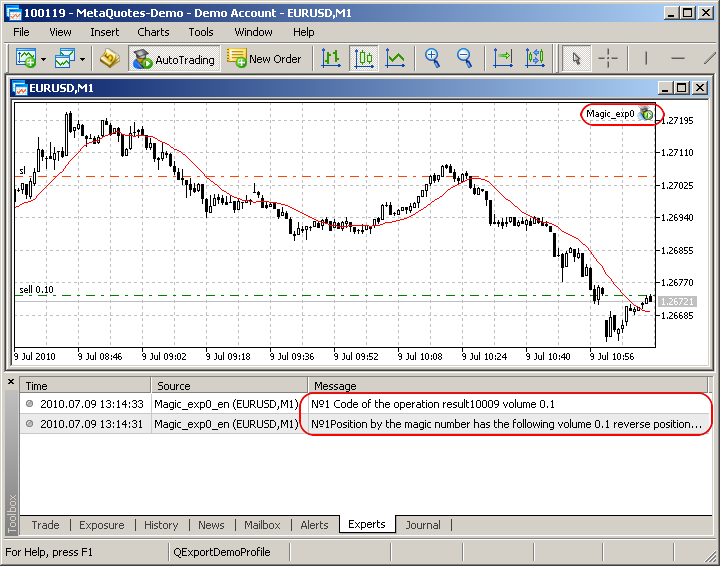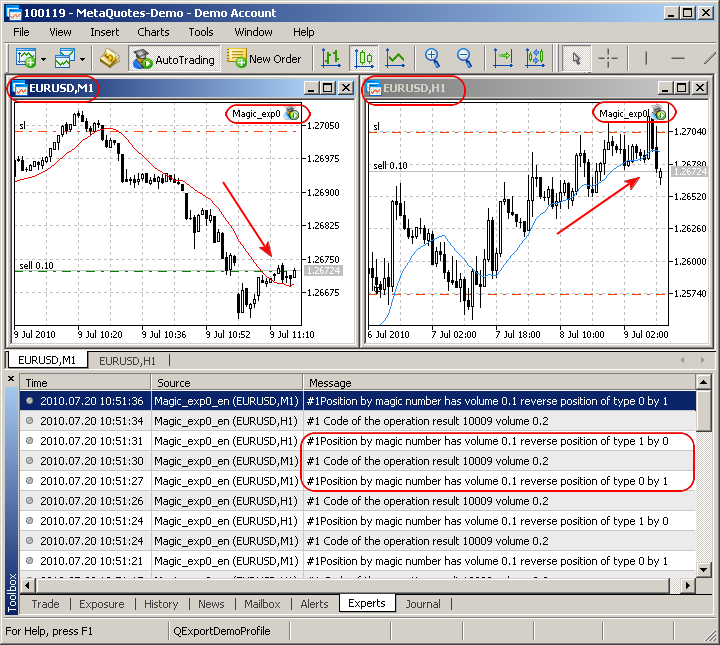### 仓位或虚拟仓位？

```// 返回开仓数量.
int     PositionsTotal();

// 返回开仓列表中相应号码位置的交易品种.
string  PositionGetSymbol(int  index);

// 选择开仓用于将来工作.
bool    PositionSelect(string  symbol, uint timeout=0);

// 函数返回开仓的请求属性.
double  PositionGetDouble(ENUM_POSITION_PROPERTY  property_id);

// 函数返回开仓的请求属性.
long    PositionGetInteger(ENUM_POSITION_PROPERTY  property_id);

// 函数返回开仓的请求属性.
string  PositionGetString(ENUM_POSITION_PROPERTY  property_id);

```

 标识符 说明 类型 POSITION_VOLUME 持仓量 double POSITION_PRICE_OPEN 持仓价 double POSITION_SL 未平仓位的止损价 double POSITION_TP 未平仓位的获利价 double POSITION_PRICE_CURRENT 交易品种的当前价 double POSITION_COMMISSION 手续费 double POSITION_SWAP 累积掉期 double POSITION_PROFIT 当前盈利 double

 标识符 说明 类型 POSITION_TIME 建仓时间 datetime POSITION_TYPE 仓位类型 ENUM_POSITION_TYPE POSITION_MAGIC 仓位的幻数（参见 ORDER_MAGIC） long POSITION_IDENTIFIER 仓位的标识 - 这是指定给重新建立的仓位的唯一数字，在其整个寿命期间不会改变。仓位的交易量不会改变其 ID。 long

 标识符 说明 类型 POSITION_SYMBOL 为其建仓的交易品种 string POSITION_COMMENT 仓位注释 string

```//+------------------------------------------------------------------
//| CPositionVirtualMagic 类结构                                      |
//+------------------------------------------------------------------
struct SPositionVirtualMagic
{
double            volume; // 虚拟仓位持仓量
ENUM_POSITION_TYPE type;  // 虚拟仓位类型
};
//+--------------------------------------------------------------------------------+
//| 本类依 EA 的魔幻号计算虚拟仓位                                                     |
//+--------------------------------------------------------------------------------+
class CPositionVirtualMagic
{
protected:
SPositionVirtualMagic pvm;
public:
double               cVOLUME(){return(pvm.volume);}
// 返回 EA 的虚拟仓位持仓量
ENUM_POSITION_TYPE   cTYPE(){return(pvm.type);}
// 返回 EA 的虚拟仓位类型
bool              PositionVirtualMagic(ulong Magic,
string symbol,
datetime CurrentTime
);
// 虚拟仓位的计算方法 返回虚拟仓位存在或不存在.
private:
void              prHistory_Deals(ulong &buf[],int HTD);
// 填充单号数组
};
//+-------------------------------------------------------------------------------------+
//| 虚拟仓位计算方法, 返回 true 如果有虚拟仓位.                                              |
//+-------------------------------------------------------------------------------------+
bool  CPositionVirtualMagic::PositionVirtualMagic(ulong Magic,
string symbol,
datetime CurrentTime
)
{
int DIGITS=(int)-log10(SymbolInfoDouble(symbol,SYMBOL_VOLUME_STEP));
if(DIGITS<0)DIGITS=0;
ulong Dticket=0;
int History_Total_Deals=-1;
ulong DTicketbuf[];

do
{
if(HistorySelect(0,TimeCurrent()))
{
History_Total_Deals=HistoryDealsTotal();
prHistory_Deals(DTicketbuf,History_Total_Deals);
}
HistorySelect(0,TimeCurrent());
}
while(History_Total_Deals!=HistoryDealsTotal());

for(int t=0;t<History_Total_Deals;t++)
{
Dticket=DTicketbuf[t];
if(HistoryDealSelect(Dticket))
{
if(HistoryDealGetInteger(Dticket,DEAL_TIME)>=CurrentTime)
{
if(HistoryDealGetInteger(Dticket,DEAL_MAGIC)==Magic)
{
{
}
else
{
if(HistoryDealGetInteger(Dticket,DEAL_TYPE)==DEAL_TYPE_SELL)
{
volume_SELL+=HistoryDealGetDouble(Dticket,DEAL_VOLUME);
}
}
}
}
}
else{HistorySelect(0,TimeCurrent());t--;}
// 如果失败, 加载历史数据并再次传递到此步
}
if(volume<0)pvm.type=POSITION_TYPE_SELL;
else
{
}
pvm.volume=fabs(volume);
if(pvm.volume==0)return(false);
else return(true);
}
```

`struct SPositionVirtualMagic`

```double               cVOLUME(){return(pvm.volume);} // 返回 EA 的虚拟仓位持仓量
ENUM_POSITION_TYPE   cTYPE()  {return(pvm.type);}   // 返回 EA 的虚拟仓位类型
```

`bool              PositionVirtualMagic(ulong Magic,string symbol,datetime CurrentTime);`

`void              prHistory_Deals(ulong &buf[],int HTD);`

`int DIGITS=(int)-log10(SymbolInfoDouble(symbol,SYMBOL_VOLUME_STEP)); if(DIGITS<0)DIGITS=0;`

```    do
{
if(HistorySelect(0,TimeCurrent()))
{
History_Total_Deals=HistoryDealsTotal();
prHistory_Deals(DTicketbuf,History_Total_Deals);
}
HistorySelect(0,TimeCurrent());
}
while(History_Total_Deals!=HistoryDealsTotal());
```

`HistorySelect(0,TimeCurrent())`

`CurrentTime = TimeCurrent();`

`History_Total_Deals=HistoryDealsTotal();`

`while(History_Total_Deals!=HistoryDealsTotal());`

`volume=NormalizeDouble(volume_BUY-volume_SELL,DIGITS);`

```   if(volume<0)pvm.type=POSITION_TYPE_SELL;
else
{
}
```

`pvm.volume=fabs(volume);`

函数值的输出：如果持仓量为 0，则其为 false，如果存在持仓量，则其为 true：

```   if(pvm.volume==0)return(false);
else return(true);
```

```//+------------------------------------------------------------------
//| EA 代码                                                          |
//+------------------------------------------------------------------

//--- 输入参数
input ulong              magic       =1;           // 标识号
input int                SL          =300;         // 止损
input int                TP          =1000;        // 获利
input int                MA_Period   =25;          // MA 周期
input double             lot         =0.1;         // 仓位持仓量
input int                MA_shift    =0;          // 指标偏移
input ENUM_MA_METHOD     MA_smooth   =MODE_SMA;     // 平滑类型
input ENUM_APPLIED_PRICE price       =PRICE_OPEN;    // 价格类型
//--- 我们将保存指标句柄
int MA_handle,type_MA,rezult;
double v;
datetime  CurrentTime;  // 保存 EA 开始时间的变量
MqlTick          tick;  // 最后市场信息结构的指针
CPositionVirtualMagic cpvm;
CProvision prov;
//+------------------------------------------------------------------
//| EA 初始化函数                                                     |
//+------------------------------------------------------------------
int OnInit()
{
CurrentTime=TimeCurrent();// 保存 EA 开始时间的变量
//--- 创建指标的句柄
MA_handle=iMA(Symbol(),0,MA_Period,MA_shift,MA_smooth,price);
return(0);
}
//+------------------------------------------------------------------
//| EA 即时价格处理函数                                                |
//+------------------------------------------------------------------
void OnTick()
{
if(CopyBuffer(MA_handle,0,0,2,v)<=0)
{Print("#",magic,"复制错误");return;}
type_MA=prov.TYPE(v); // 依据 MA 指示判断类型

if(cpvm.PositionVirtualMagic(magic,_Symbol,CurrentTime))// 如果此时有开仓
{
if((int)cpvm.cTYPE()!=type_MA)// 检查是否是平仓时机
{
Print("#",magic,"标识号对应的仓位持仓量 ",cpvm.cVOLUME(),
" 反向仓位类型 ",(int)cpvm.cTYPE()," 依据 ",type_MA);
//cpvm.cVOLUME() - 虚拟仓位持仓量
rezult=prov.SendOrder((ENUM_ORDER_TYPE)type_MA,cpvm.cVOLUME()+lot);// 反向仓位
if(rezult!=-1)Print("#",magic," 操作结果代码 ",rezult," 交易量 ",res.volume);
else{Print("#",magic,"错误",GetLastError()); return;}
}
}
else // 如果没有仓位则开仓
{
Print("#",magic,"标识号对应的仓位持仓量 ",cpvm.cVOLUME()," 仓位类型 ",type_MA);
rezult=prov.SendOrder((ENUM_ORDER_TYPE)type_MA,lot);// 开仓
if(rezult!=-1)Print("#",magic," 操作结果代码 ",rezult," 交易量 ",res.volume);
else{Print("#",magic,"错误",GetLastError()); return;}
}
}
```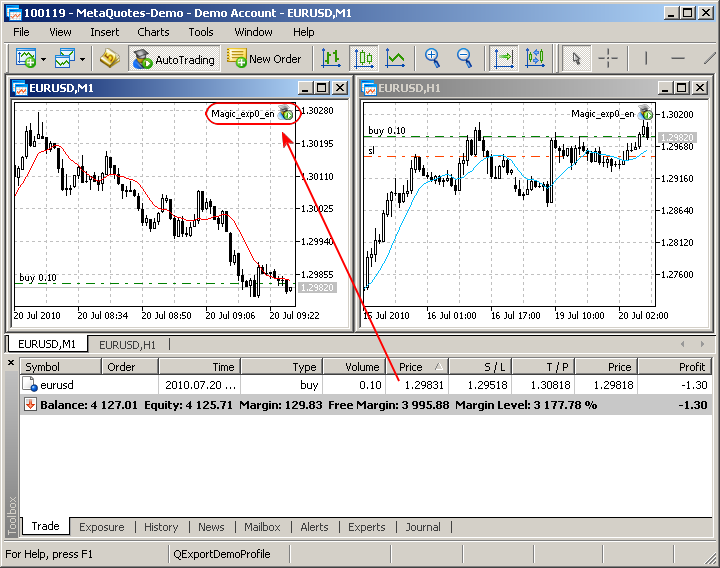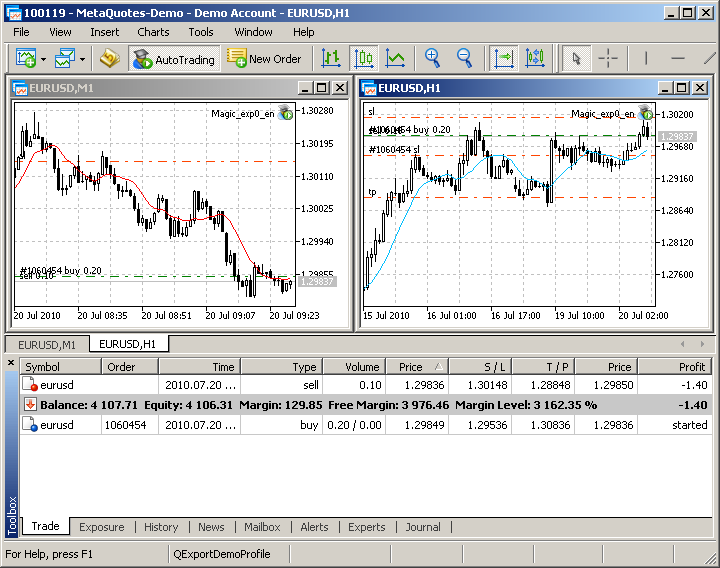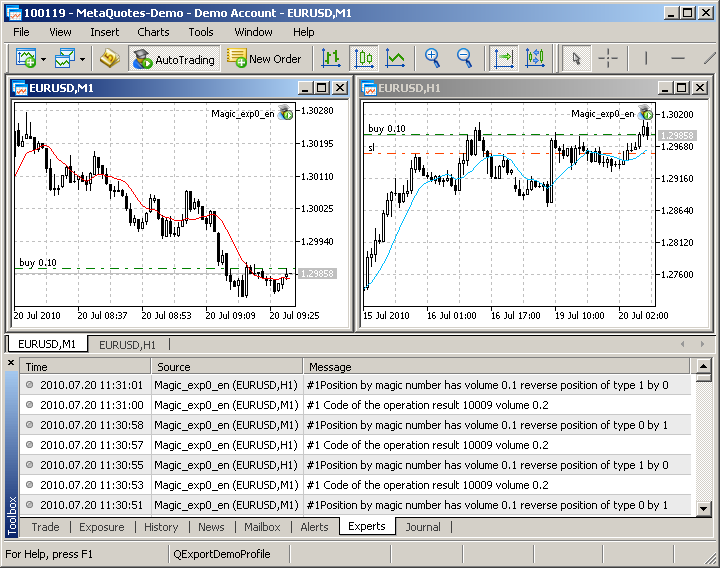### 对幻数编码

• 方法需要对 EA 交易的名称进行编码（让我们将其称为数字名称）
• 识别“您的/外来的”代码（让我们将其称为互动代码）
• 交易品种代码，EA 交易处理的交易品种（为了能够从交易确定 EA 交易是从哪里工作的）

```enum Emagic
{
ENUM_DIGITAL_NAME,    // EA 数字名称
ENUM_CODE_INTERACTION,// 交互代码
ENUM_EXPERT_SYMBOL    // EA 启动时的交易品种
};
```

```struct Smagic
{
ulong             magicnumber;      // 幻数是一种组装形式 - 它依顺序写
int               digital_name;     // 数字名
int               code_interaction; // 交互代码
int               expert_symbol;    // EA 启动时的交易品种
};
```

```class Cmagic
{
protected:
Smagic            mag;
SPositionVirtualMagic pvm;
public:
// 函数返回组装的幻数, 依输入数据组装
ulong             SetMagic_request(int digital_name=0,int code_interaction=0);

// 函数获取组装的幻数并按组装逻辑拆分
ulong             SetMagic_result(ulong magicnumber);

// 函数获取返回识别并返回组装幻数的请求部分
ulong             GetMagic_result(Emagic enum_);

// 函数获取返回的标识符 并返回组装幻数的请求部分文本解释
string            sGetMagic_result(Emagic enum_);

// 返回 EA 的虚拟仓位持仓量
double            cVOLUME(){return(pvm.volume);}

// 返回 EA 的虚拟仓位类型
ENUM_POSITION_TYPE  cTYPE(){return(pvm.type);}

// 虚拟仓位计算方法, 返回虚拟仓位存在或不存在
bool              PositionVirtualMagic(Emagic enum_,
string symbol,
datetime CurrentTime);
private:
// 函数拆分幻数为三部分  并返回指定类别的部分
int               decodeMagic_result(int category);

// 金融工具交易品种的解释器对应数字代码
int               symbolexpert();

// 数字代码的解释器对应的预描述文本 (EA)
string            expertcode(int code);

// 数字代码的解释器对应的预描述文本 (交互)
string            codeinterdescript(int code);

// 数字代码的解释器对应的金融工具交易品种
string            symbolexpert(int code);

// 记录单号周期至缓存区
void              prHistory_Deals(ulong &buf[],int HTD);
};
```

```//+------------------------------------------------------------------
//| 函数返回组装的幻数, 依输入数据组装                                   |
//+------------------------------------------------------------------
ulong Cmagic::SetMagic_request(int digital_name=0,int code_interaction=0)
{
if(digital_name>=1000)Print("指定 EA 数字名称不正确 (超过 1000)");
if(code_interaction>=1000)Print("指定识别代码不正确 (超过 1000)");
mag.digital_name     =digital_name;
mag.code_interaction =code_interaction;
mag.expert_symbol    =symbolexpert();
mag.magicnumber      =mag.digital_name*(int)pow(1000,2)+
mag.code_interaction*(int)pow(1000,1)+
mag.expert_symbol;
return(mag.magicnumber);
}
```

`ulong Cmagic::SetMagic_request(int digital_name=0,int code_interaction=0)`

```   if(digital_name>=1000)Print("指定 EA 数字名称不正确 (超过 1000)");
if(code_interaction>=1000)Print("指定识别代码不正确 (超过 1000)");
```

`int Cmagic::symbolexpert()`

```mag.magicnumber      =mag.digital_name*(int)pow(1000,2)+
mag.code_interaction*(int)pow(1000,1)+
mag.expert_symbol;
```

```//+------------------------------------------------------------------
//| 函数获取组装的幻数                                                 |
//| 并按组装逻辑拆分                                                   |
//+------------------------------------------------------------------
ulong Cmagic::SetMagic_result(ulong magicnumber)
{
mag.magicnumber      =magicnumber;
mag.expert_symbol    =decodeMagic_result(1);
mag.code_interaction =decodeMagic_result(2);
mag.digital_name     =decodeMagic_result(3);
return(mag.magicnumber);
}
```

```//+------------------------------------------------------------------
//| 函数拆分魔幻号为三部分                                              |
//| 并返回指定类别的部分                                               |
//+------------------------------------------------------------------
int Cmagic::decodeMagic_result(int category)
{
string string_value=(string)mag.magicnumber;
int rem=(int)MathMod(StringLen(string_value),3);
if(rem!=0)
{
rem=3-rem;
string srem="0";
if(rem==2)srem="00";
string_value=srem+string_value;
}
int start_pos=StringLen(string_value)-3*category;
string value=StringSubstr(string_value,start_pos,3);
return((int)StringToInteger(value));
}
```

```string string_value=(string)mag.magicnumber;

```

```//+------------------------------------------------------------------
//| 函数获取返回的标识符                                               |
//| 并返回组装幻数的请求部分                                            |
//+------------------------------------------------------------------
ulong Cmagic::GetMagic_result(Emagic enum_)
{
switch(enum_)
{
case ENUM_DIGITAL_NAME     : return(mag.digital_name);     break;
case ENUM_CODE_INTERACTION : return(mag.code_interaction); break;
case ENUM_EXPERT_SYMBOL    : return(mag.expert_symbol);    break;
default: return(mag.magicnumber); break;
}
}
//+------------------------------------------------------------------------------+
//| 函数获取返回的标识符并返回                                                       |
//| 组装幻数的请求部分文本解释                                                       |
//+------------------------------------------------------------------------------+
string Cmagic::sGetMagic_result(Emagic enum_)
{
switch(enum_)
{
case ENUM_DIGITAL_NAME     : return(expertcode(mag.digital_name));            break;
case ENUM_CODE_INTERACTION : return(codeinterdescript(mag.code_interaction)); break;
case ENUM_EXPERT_SYMBOL    : return(symbolexpert(mag.expert_symbol));         break;
default: return((string)mag.magicnumber); break;
}
}
```

```bool  Cmagic::PositionVirtualMagic(Emagic enum_,
string symbol,
datetime CurrentTime)
```

```//+------------------------------------------------------------------
//| EA 代码                                                          |
//+------------------------------------------------------------------
//--- 输入参数
input ulong              digital_name_       =4;           // EA 数字名称
input ulong              code_interaction_   =1;           // 交互代码
input Emagic             _enum               =0;           // 幻数模式
input int                SL                  =300;         // 止损
input int                TP                  =1000;        // 获利
input int                MA_Period           =25;          // MA 周期
input double             lot                 =0.4;         // 仓位持仓量
input int                MA_shift            =0;           // 指标偏移
input ENUM_MA_METHOD     MA_smooth           =MODE_SMA;      // 平滑类型
input ENUM_APPLIED_PRICE price               =PRICE_OPEN;    // 价格类型

//--- 我们将保存指标句柄
int MA_handle,type_MA,rezult;
static ulong magic;
double v;
datetime  CurrentTime;// 保存 EA 开始时间的变量

MqlTick         tick;  // 最后市场信息结构的指针
CProvision prov;
Cmagic mg;
//+------------------------------------------------------------------
//| EA 初始化函数                                                     |
//+------------------------------------------------------------------
int OnInit()
{
magic=mg.SetMagic_request(digital_name_,code_interaction_);
// EA 印记 (幻数标识符), 此标识符声明作为全局变量
// 用于 int CProvision::SendOrder(ENUM_ORDER_TYPE type,double volume)
CurrentTime=TimeCurrent();// 保存 EA 开始时间的变量
//--- 创建指标的句柄
MA_handle=iMA(Symbol(),0,MA_Period,MA_shift,MA_smooth,price);
return(0);
}
//+------------------------------------------------------------------
//| EA 即时价格处理函数                                                |
//+------------------------------------------------------------------
void OnTick()
{
if(CopyBuffer(MA_handle,0,0,2,v)<=0)
{Print("#",magic,"复制错误");return;}
type_MA=prov.TYPE(v); // 依据 MA 指示判断类型
mg.SetMagic_result(magic);// 将信息放于结构
if(mg.PositionVirtualMagic(_enum,_Symbol,CurrentTime))// 如果有开仓
{
if((int)mg.cTYPE()!=type_MA)// 检查是否是平仓时机
{
mg.SetMagic_result(magic);// 将信息放于结构
Print("#",mg.GetMagic_result(_enum),"标识号对应的仓位持仓量 ",mg.cVOLUME(),
" 反向仓位类型 ",(int)mg.cTYPE()," 依据 ",type_MA);
//cpvm.cVOLUME() - 虚拟仓位持仓量
rezult=prov.SendOrder((ENUM_ORDER_TYPE)type_MA,mg.cVOLUME()+lot);// 反向仓位
if(rezult!=-1)Print("№",magic," 操作结果代码 ",rezult," 交易量 ",res.volume);
else{Print("№",magic,"错误",GetLastError()); return;}
}
}
else // 如果没有仓位则开仓
{
Print("#",magic,"标识号对应的仓位持仓量   ",mg.cVOLUME()," 仓位类型",type_MA);
rezult=prov.SendOrder((ENUM_ORDER_TYPE)type_MA,lot);// 开仓
if(rezult!=-1)Print("#",magic," 操作结果代码 ",rezult," 交易量 ",res.volume);
else{Print("#",magic,"错误",GetLastError()); return;}
}
}
```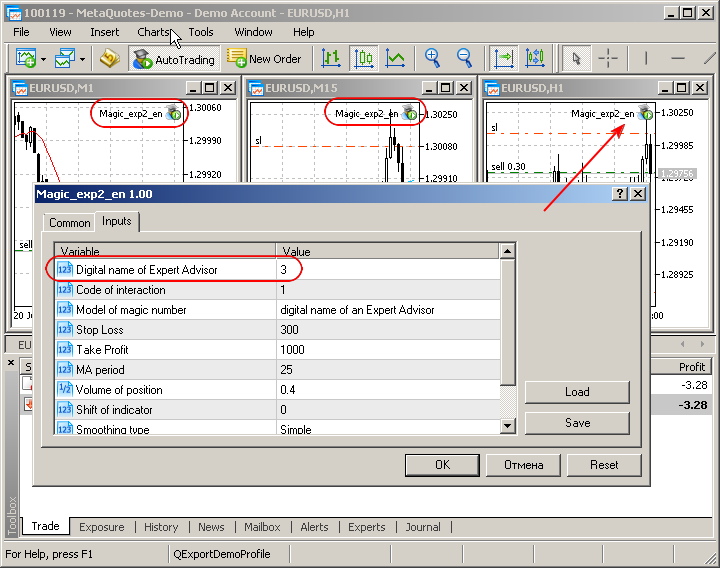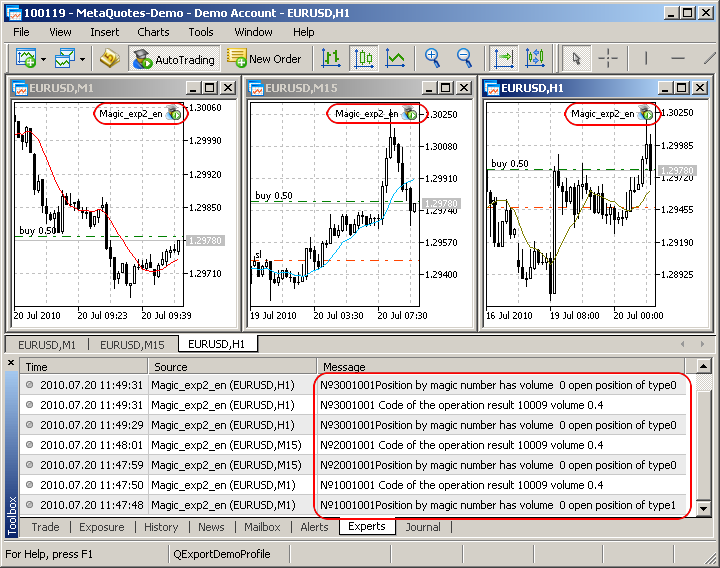### 总结

magic_exp0_en.mq5 (14.33 KB)
magic_exp1_en.mq5 (21.03 KB)
magic_exp2_en.mq5 (45.74 KB)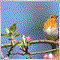| 6 12月 2013 在 14:11
MetaQuotes:
Great   ！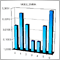中测试移动平均线计算的性能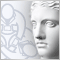用 Delphi 为 MQL5 编写 DLL 指南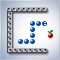用 MQL5 创建“贪吃蛇”游戏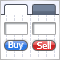用 MQL5 创建交易活动控制板